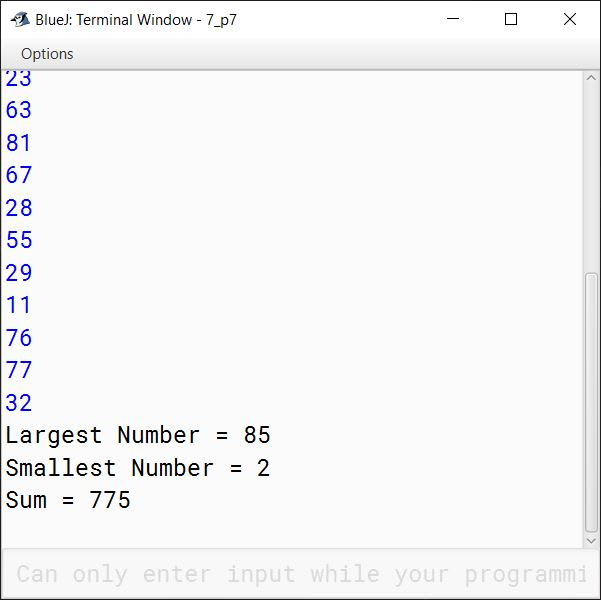# Solved Sample Paper 1

## Section A

#### Question 1

(a) Define Java byte code.

Java compiler converts Java source code into an intermediate binary code called Bytecode. Bytecode can't be executed directly on the processor. It needs to be converted into Machine Code first.

(b) Write a difference between class and an object.

A class is a blue print that represents a set of objects that share common characteristics and behaviour whereas an object is a specific instance of a class having a specific identity, specific characteristics and specific behaviour.

(c) Name the following:

1. The keyword which converts variable into constant.
2. The method which terminates the entire program from any stage.

1. final
2. System.exit(0)

(d) Which of the following are primitive data types?

1. double
2. String
3. char
4. Integer

1. Primitive
2. Non-Primitive
3. Primitive
4. Non-Primitive

(e) What is an operator? What are the three main types of operators? Name them.

An operator is a symbol or sign used to specify an operation to be performed in Java programming. The three main types of operators are Arithmetical, Logical and Relational.

#### Question 2

(a) What is Autoboxing in Java? Give an example.

The automatic conversion of primitive data type into an object of its equivalent wrapper class is known as Autoboxing. For example, the below statement shows the conversion of an int to an Integer.

Integer a = 20;

(b) Differentiate between length and length().

lengthlength()
length is an attribute i.e. a data member of array.length() is a member method of String class.
It gives the length of an array i.e. the number of elements stored in an array.It gives the number of characters present in a string.

Constructor overloading is a technique in Java through which a class can have more than one constructor with different parameter lists. The different constructors of the class are differentiated by the compiler using the number of parameters in the list and their types. For example, a class Employee can have 3 constructors as shown below:

Employee(long empId)
Employee(String name, double salary)
Employee(long empId, String name)

Due to constructor overloading, all the 3 constructors are valid for Employee class.

(d) What is the use of the keyword 'import' in Java programming?

import keyword is used to import built-in and user-defined packages into our Java program.

(e) What is meant by an infinite loop? Give an example.

A loop which continues iterating indefinitely and never stops is termed as infinite loop. Below is an example of infinite loop:

for (;;)
System.out.println("Infinite Loop");

#### Question 3

(a) Write a Java expression for the following:

$\sqrt{b^2 - 4ac}$

Math.sqrt(b * b - 4 * a * c)

(b) Evaluate the following if the value of x=7, y=5

x += x++ + x + ++y

x += x++ + x + ++y
⇒ x = x + (x++ + x + ++y)
⇒ x = 7 + (7 + 8 + 6)
⇒ x = 7 + 21
⇒ x = 28

(c) Write the output for the following:

String s1 = "Life is Beautiful";
System.out.println("Earth" + s1.substring(4));
System.out.println(s1.endsWith("L"));

##### Output
Earth is Beautiful
false

##### Explanation

s1.substring(4) will return a substring starting at index 4 of s1 till its end so its output will be " is Beautiful". System.out.println("Earth" + s1.substring(4)) will concatenate "Earth" and " is Beautiful" to given the output as "Earth is Beautiful".

As the last character of s1 is a small l not a capital L so s1.endsWith("L") returns false.

(d) Write the output of the following statement:

System.out.println("A picture is worth \t \"A thousand words.\" ");

##### Output
A picture is worth       "A thousand words."

##### Explanation

\t is the escape sequence for tab. \" is used to print quotes as part of the string.

(e) Give the output of the following program segment and mention how many times the loop will execute:

int k;
for ( k = 5 ; k < = 20 ; k + = 7 )
if ( k% 6==0 )
continue;
System.out.println(k);

Output of the program is 26 and the loop executes 3 times.

As there are no curly braces after the for loop so only the statement immediately following the loop that is the if statement is part of the for loop. Again if has no curly braces so just the continue statement is part of if. The statement System.out.println(k) is outside the loop. The below table shows each iteration of for loop in detail:

kRemarks
51st Iteration
122nd Iteration
193rd Iteration
26As the loop condition k<=20 becomes false, the loop stops iterating after 3 iterations.

(f) What is the data type returned by the following library methods?

1. isWhitespace()
2. compareToIgnoreCase()

1. boolean
2. int

(g) Rewrite the following program segment using logical operators:

if ( x > 5 )
if ( x > y )
System.out.println(x+y);

if ( x > 5 && x > y)
System.out.println(x+y);

(h) Convert the following if else if construct into switch case:

if (ch== 'c' || ch=='C')
System.out.print("COMPUTER");
else if (ch == 'h' || ch =='H')
System.out.print("HINDI");
else
System.out.print("PHYSICAL EDUCATION");

switch (ch) {
case 'c':
case 'C':
System.out.print("COMPUTER");
break;
case 'h':
case 'H':
System.out.print("HINDI");
break;
default:
System.out.print("PHYSICAL EDUCATION");

}

(i) Give the output of the following:

1. Math.pow(36,0.5) + Math.cbrt(125)
2. Math.ceil(4.2) + Math.floor(7.9)

1. 11.0
2. 12.0
##### Working

Math.pow(36,0.5) gives 6.0 and Math.cbrt(125) gives 5.0. 6.0 + 5.0 = 11.0

Math.ceil(4.2) gives 5.0 and Math.floor(7.9) gives 7.0. 5.0 + 7.0 = 12.0.

(j) Rewrite the following using ternary operator:

if(n1>n2)
r = true;
else
r = false;

r = n1 > n2 ? true : false;

## Section B

#### Question 4

A private Cab service company provides service within the city at the following rates:

AC CARNON AC CAR
Upto 5 KM₹150/-₹120/-
Beyond 5 KM₹10/- PER KM₹08/- PER KM

Design a class CabService with the following description:

Member variables /data members:

String car_type — To store the type of car (AC or NON AC)

double km — To store the kilometer travelled

double bill — To calculate and store the bill amount

Member methods:

CabService() — Default constructor to initialize data members. String data members to '' '' and double data members to 0.0.

void accept() — To accept car_type and km (using Scanner class only).

void calculate() — To calculate the bill as per the rules given above.

void display() — To display the bill as per the following format:

CAR TYPE:
KILOMETER TRAVELLED:
TOTAL BILL:

Create an object of the class in the main method and invoke the member methods.

import java.util.Scanner;

public class CabService
{
String car_type;
double km;
double bill;

public CabService() {
car_type = "";
km = 0.0;
bill = 0.0;
}

public void accept() {
Scanner in = new Scanner(System.in);
System.out.print("Enter car type: ");
car_type = in.nextLine();
System.out.print("Enter kilometer: ");
km = in.nextDouble();
}

public void calculate() {
if (km <= 5) {
if (car_type.equals("AC"))
bill = 150;
else
bill = 120;
}
else {
if (car_type.equals("AC"))
bill = 150 + 10 * (km - 5);
else
bill = 120 + 8 * (km - 5);
}
}

public void display() {
System.out.println("Car Type: " + car_type);
System.out.println("Kilometer Travelled: " + km);
System.out.println("Total Bill: " + bill);
}

public static void main(String args[]) {
CabService obj = new CabService();
obj.accept();
obj.calculate();
obj.display();
}
}
##### Output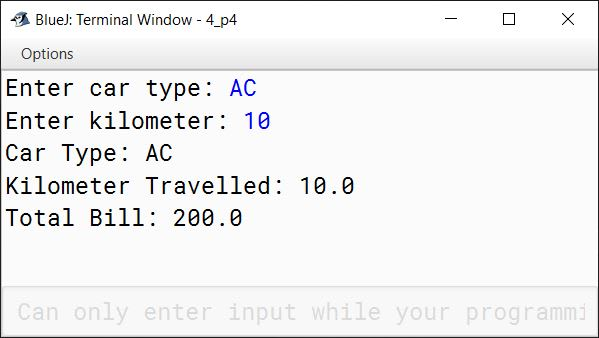#### Question 5

Write a program to search for an integer value input by the user in the list given below using linear search technique. If found display "Search Successful" and print the index of the element in the array, otherwise display "Search Unsuccessful".

{75, 86, 90, 45, 31, 50, 36, 60, 12, 47}

import java.util.Scanner;

public class KboatLinearSearch
{
public static void main(String args[]) {
Scanner in = new Scanner(System.in);

int arr[] = {75, 86, 90, 45, 31, 50, 36, 60, 12, 47};
int l = arr.length;
int i = 0;

System.out.print("Enter the number to search: ");
int n = in.nextInt();

for (i = 0; i < l; i++) {
if (arr[i] == n) {
break;
}
}

if (i == l) {
System.out.println("Search Unsuccessful");
}
else {
System.out.println("Search Successful");
System.out.println(n + " present at index " + i);
}
}
}
##### Output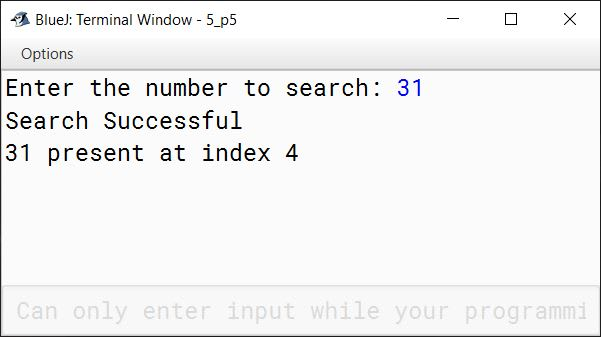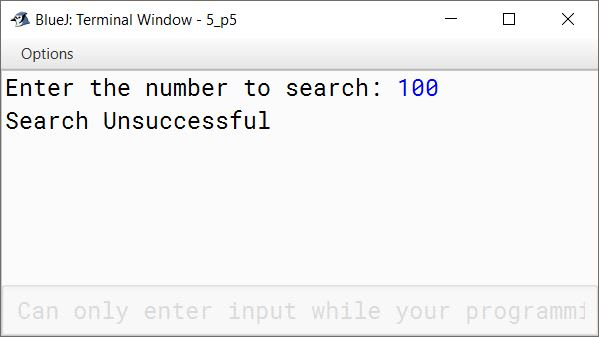#### Question 6

An Abundant number is a number for which the sum of its proper factors is greater than the number itself. Write a program to input a number and check and print whether it is an Abundant number or not.

Example:

Consider the number 12.

Factors of 12 = 1, 2, 3, 4, 6 Sum of factors = 1 + 2 + 3 + 4 + 6 = 16

As 16 > 12 so 12 is an Abundant number.

import java.util.Scanner;

public class KboatAbundantNumber
{
public static void main(String args[]) {
Scanner in = new Scanner(System.in);
System.out.print("Enter the number: ");
int n = in.nextInt();
int sum = 0;

for (int i = 1; i < n; i++) {
if (n % i == 0)
sum += i;
}

if (sum > n)
System.out.println(n + " is an abundant number");
else
System.out.println(n + " is not an abundant number");
}
}
##### Output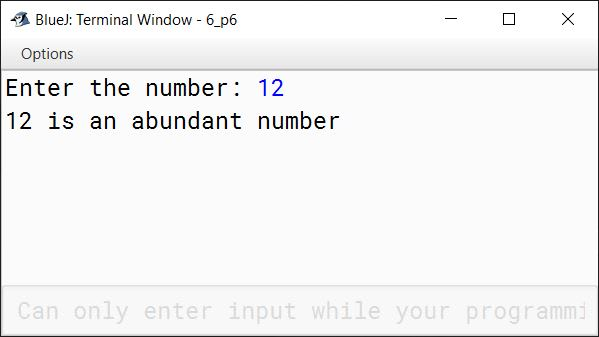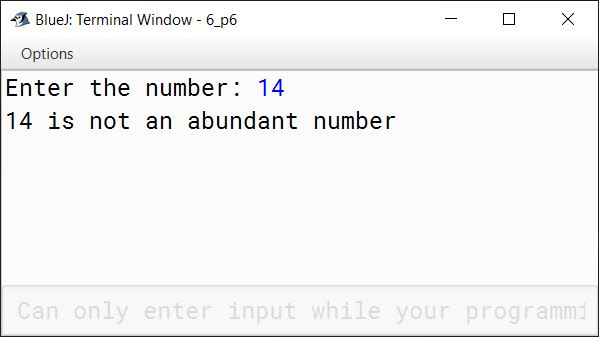#### Question 7

Design a class to overload a method Number() as follows:

(i) void Number(int num, int d) — To count and display the frequency of a digit in a number.

Example:

num = 2565685
d = 5
Frequency of digit 5 = 3

(ii) void Number(int n1) — To find and display the sum of even digits of a number.

Example:

n1 = 29865
Sum of even digits = 2 + 8 + 6 = 16

Write a main method to create an object and invoke the above methods.

public class Overload
{
void Number(int num, int d) {
int f = 0;

while (num != 0) {
int x = num % 10;
if (x == d) {
f++;
}
num /= 10;
}

System.out.println("Frequency of digit " + d + " = " + f);
}

void Number(int n1) {
int s = 0;

while (n1 != 0) {
int x = n1 % 10;
if (x % 2 == 0) {
s = s + x;
}
n1 /= 10;
}

System.out.println("Sum of even digits = " + s);
}

public static void main(String args[]) {
obj.Number(2565685, 5);
obj.Number(29865);
}
}
##### Output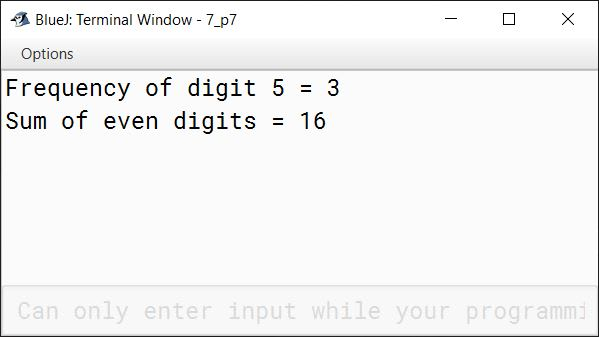#### Question 8

Write a menu driven program to perform the following operations as per user’s choice:

(i) To print the value of c=a²+2ab, where a varies from 1.0 to 20.0 with increment of 2.0 and b=3.0 is a constant.

(ii) To display the following pattern using for loop:

A
AB
ABC
ABCD
ABCDE

Display proper message for an invalid choice.

import java.util.Scanner;

{
public static void main(String args[]) {
Scanner in = new Scanner(System.in);
System.out.println("Type 1 for Value of c");
System.out.println("Type 2 for pattern");
System.out.print("Enter your choice: ");

int choice = in.nextInt();

switch (choice) {
case 1:
final double b = 3.0;
for (int a = 1; a <= 20; a += 2) {
double c = Math.pow(a, 2) + 2 * a * b;
System.out.println("Value of c when a is "
+ a + " = " + c);
}
break;

case 2:
for (char i = 'A'; i <= 'E'; i++) {
for (char j = 'A'; j <= i; j++) {
System.out.print(j);
}
System.out.println();
}
break;

default:
System.out.println("INVALID CHOICE");
}
}
}
##### Output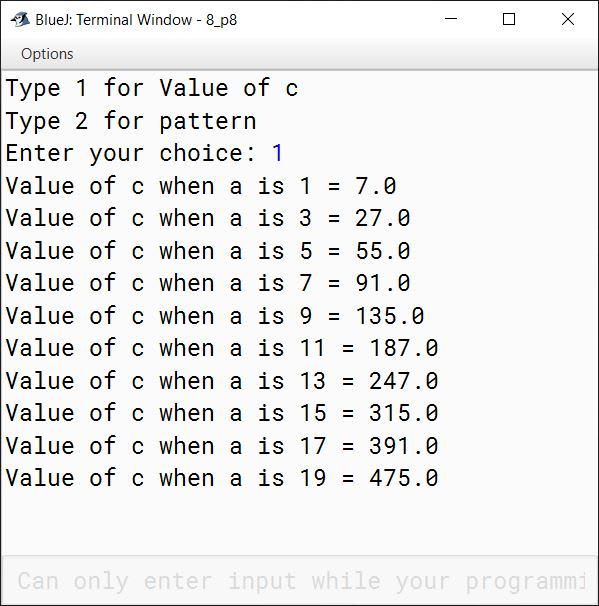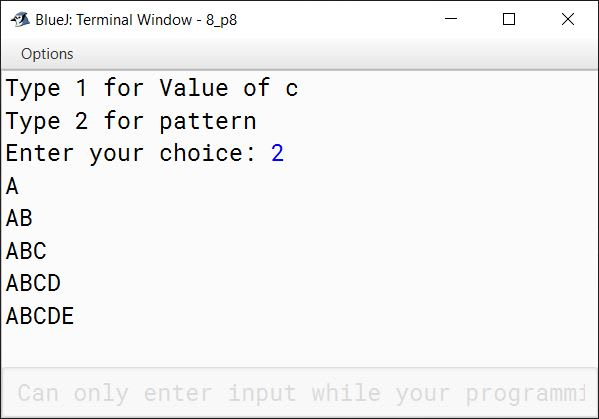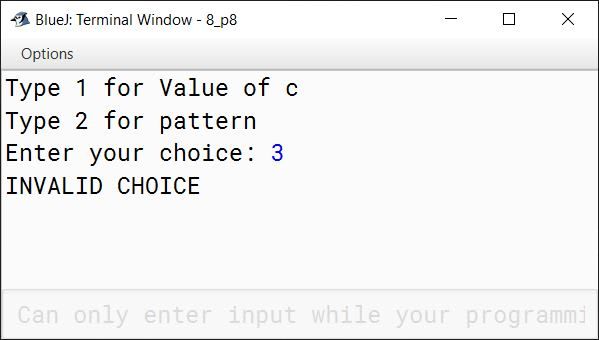#### Question 9

Write a program to input integer elements into an array of size 20 and perform the following operations:

1. Display largest number from the array.
2. Display smallest number from the array.
3. Display sum of all the elements of the array

import java.util.Scanner;

public class KboatSDAMinMaxSum
{
public static void main(String args[]) {
Scanner in = new Scanner(System.in);
int arr[] = new int;
System.out.println("Enter 20 numbers:");
for (int i = 0; i < 20; i++) {
arr[i] = in.nextInt();
}
int min = arr, max = arr, sum = 0;
for (int i = 0; i < arr.length; i++) {
if (arr[i] < min)
min = arr[i];

if (arr[i] > max)
max = arr[i];

sum += arr[i];
}

System.out.println("Largest Number = " + max);
System.out.println("Smallest Number = " + min);
System.out.println("Sum = " + sum);
}
}
##### Output